## Numerical - Multi Cylinder Inline Engine (with firing order)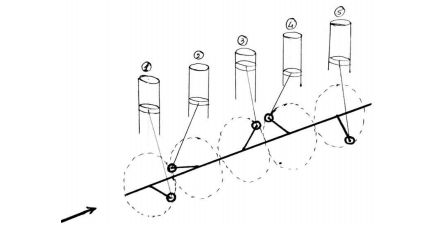fig. 1

## Basic knowledge about Firing Order

The firing order is a sequence of giving spark/firing to the cylinders of multi-cylinder inline engines. Firing order is provided in multi-cylinder inline engines in order to avoid vibrations (If firing order is not provided in multi-cylinder inline engines, then all the pistons will move in upward direction at the same time and same way they move in the downward direction at the same time. This creates a lot of vibration.) For avoiding these vibrations, firing order is provided in multi-cylinder inline engines.

Numerical

### A four stroke five cylinder inline engine has a firing order of 1-4-5-3-2-1. The centre lines of cylinders are spaced at an equal interval of 15 cm, the reciprocating parts per cylinder have a mass of 1.5 kg, the piston stroke is 10 cm and the connecting rods are 17.5 cm long. The engine rotates at 600 rpm. Determine the value of maximum primary and secondary unbalanced forces and couples about the central plane.

Solution:

Given data:

\begin{aligned} \text {mass of reciprocating parts } m &= 1.5 \ kg \\ \text {Stroke } S &= 10 \ cm = 0.10 \ m \\ \text {Radius of crank } r &= {S \over 2} = {0.10 \over 2} = 0.05 \ m \\ \text {Length of connecting rod } L &= 17.5 \ cm = 0.175 \ m \\ \text {Obliquity ratio } n &= {L \over r} = {0.175 \over 0.05} = 3.5 \\ \text {Speed of engine } N &= 600 \ rpm \\ \omega &= {2 \pi N \over 60} = {2 \pi \times 600 \over 60 } = 62.85 { rad \over s} \end{aligned}

The force and couple data is given in table:

Plane Mass (m), kg Radius (r), m Centrifugal Force (m x r), kg.m Distance From R.P.(l), m Couple (m x r x l), kg.m 2 Primary Crank Position ' θ ' Secondary Crank Position '2 θ '
1 1.5 0.05 0.075 -0.3 -0.022
2 1.5 0.05 0.075 -0.15 -0.011 576° i.e. 216° 1152° i.e. 72°
3 (R.P.) 1.5 0.05 0.075 0 0 432° i.e. 72° 864° i.e. 144°
4 1.5 0.05 0.075 0.15 0.011 144° 288°
5 1.5 0.05 0.075 0.3 0.022 288° 576° i.e. 216°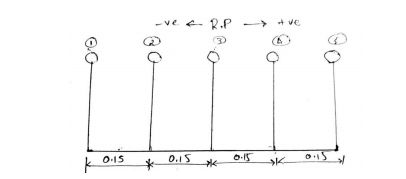fig.2 (a) Position of planes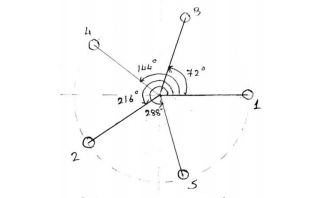fig.2 (b) Primary crank positions

• Assuming the engine to be vertical, the position of cylinders are shown in fig.2(a).
• The angles are measured from cylinder 1.
• Cylinder 3 is considered as a reference plane (R.P.)
• The engine is working on a four stroke cycle, the angle between two crank $= {720 \over 5} = 144\degree$
• Considering firing order : 1-4-5-3-2-1
\begin{aligned} \therefore \ &\theta_1 = 0\degree \\ &\theta_4 = 144\degree \\ &\theta_5 = 144\degree + 144\degree = 288\degree \\ &\theta_3 = 288\degree + 144\degree = 432\degree \\ &\theta_2 = 432\degree + 144\degree = 576\degree \end{aligned}

## 1. Primary force polygon

For drawing a primary force polygon, take a suitable scale of 1 cm = 0.075 kg.m. From fig.2(c) it is seen that, primary force polygon is closed and hence there is no unbalanced primary force.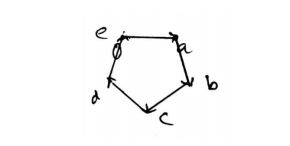fig.2 (c) Primary force polygon

## 2. Primary couple polygon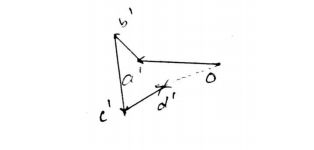fig.2 (d) Primary couple polygon

• Draw the primary couple polygon by taking a suitable scale of $1\ cm = 0.011 \ kg \ m^2$. The vector $\overrightarrow {od'}$ represents the unbalanced primary couple $C_p$.

• Now from fig.2 (d), measure $\overrightarrow {od'}$;

$\overrightarrow {od'} = 1.5 \ cm$
• Hence, the unbalanced primary couple is,
\begin{aligned} C_p &= \overrightarrow {od'} \times scale \times \omega^2 \\ &= 1.5 \times 0.011 \times (62.85)^2 \\ &= 65.18 \ N \sdot m \end{aligned}

## 3. Secondary force polygon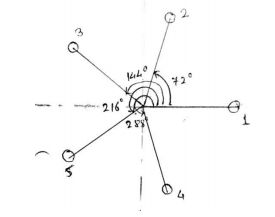fig.2 (e) Secondary crank positions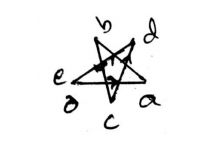fig.2 (f) Secondary force polygon

Draw the secondary force polygon by taking a suitable scale of $1 \ cm = 0.075 \ kg.m$. From fig.2 (f), it is seen that the secondary force polygon is closed, hence there is no unbalanced secondary force.

## 4. Secondary couple polygon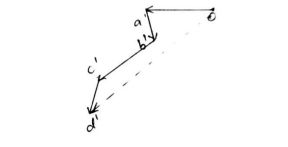fig.2 (g) Secondary couple polygon

• Draw the secondary couple polygon by taking suitable scale of $1 \ cm = 0.011 \ kg m^2$. The vector $\overrightarrow {od'}$ represents the unbalanced secondary couple ${C_s}$

• Now from fig.2 (g), measure $\overrightarrow {od'}$;

$\overrightarrow {od'} = 4.7 \ cm$
• Hence, the unbalanced secondary couple is,
\begin{aligned} C_s &= \overrightarrow {od'} \times scale \times {\omega^2 \over n} \\ &= 4.7 \times 0.011 \times {(62.85)^2 \over 3.5} \\ &= 58.3 \ N \sdot m \end{aligned}